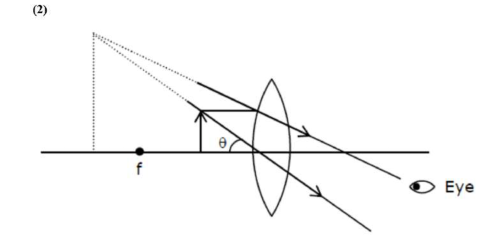# Given below are two statements: One is labeled as AssertionQuestion:

Given below are two statements: One is labeled as Assertion $A$ and the other is labeled as Reason $\mathrm{R}$. Assertion A: For a simple microscope, the angular size of the object equals the angular size of the image. Reason $\mathrm{R}$ : Magnification is achieved as the small object can be kept much closer to the eye than $25 \mathrm{~cm}$ and hence it subtends a large angle. In the light of the above statements, choose the most appropriate answer from the options given below:

1. Both $A$ and $R$ are true but $R$ is NOT the correct explanationof $A$

2. Both $\mathrm{A}$ and $\mathrm{R}$ are true and $\mathrm{R}$ is the correct explanation of $\mathrm{A}$

3. $\mathrm{A}$ is true but $\mathrm{R}$ is false

4. $\mathrm{A}$ is false but $\mathrm{R}$ is true

Correct Option: , 2

Solution:Both obtain same angle, since image can be at a distance greater than $25 \mathrm{~cm}$, object can be moved closer to eye.